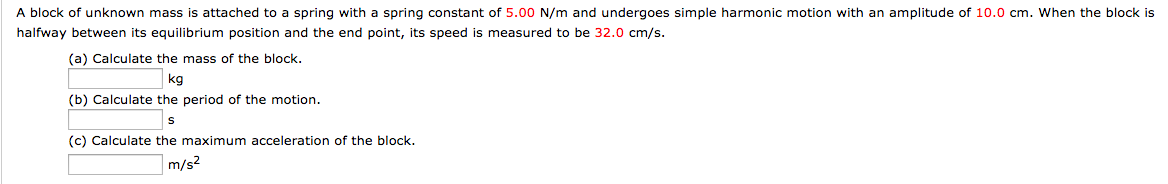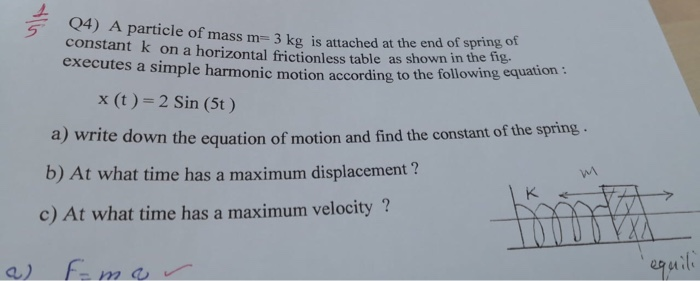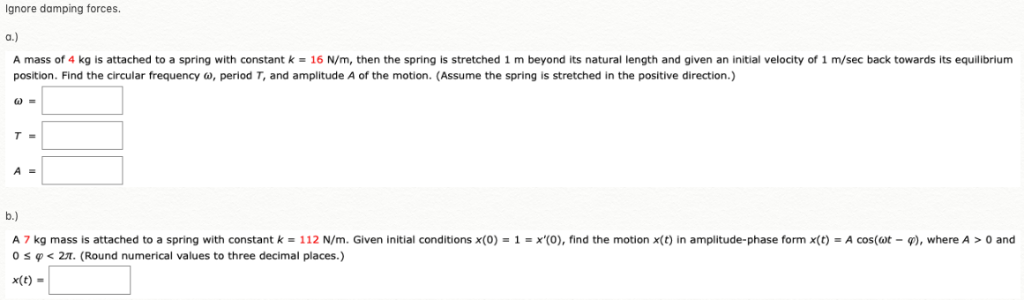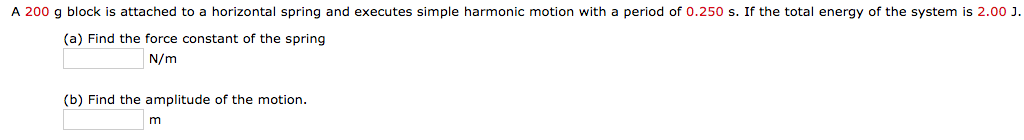# A mass of 0.160kg is attached to a spring of spring constant 41.0 N/m if the...

A mass of 0.160kg is attached to a spring of spring constant 41.0 N/m if the mass executes simple harmonic motion, what will be the period (T) of its motion ?

We know

angular frequency, w = sqrt(k/m) (here k is spring constant and m is mass)

and time periode, T = 2*pi/w

= 2*pi*sqrt(m/k)

= 2*pi*sqrt(0.16/41)

= 0.393 s

#### Earn Coin

Coins can be redeemed for fabulous gifts.

Similar Homework Help Questions
• ### A block of unknown mass is attached to a spring with a spring constant of 6.50...

A block of unknown mass is attached to a spring with a spring constant of 6.50 N/m and undergoes simple harmonic motion with an amplitude of 11.5 cm. When the block is halfway between its equilibrium position and the end point, its speed is measured to be 29.0 cm/s. (a) Calculate the mass of the block. (b) Calculate the period of the motion. (c) Calculate the maximum acceleration of the block.

• ### 2. A block of unknown mass is attached to a spring with a spring constant of...2. A block of unknown mass is attached to a spring with a spring constant of 5.00 N/m and undergoes simple harmonic motion with an amplitude of 10.0 cm. When the block is halfway between its equilibrium position and the end point, its speed is measured to be 32.0 cm/s (a) Calculate the mass of the block (b) Calculate the period of the motion (c) Calculate the maximum acceleration of the block. kg m/s

• ### Q4) A particle of mass m=3 kg is attached at the en constant kon a horizontal...Q4) A particle of mass m=3 kg is attached at the en constant kon a horizontal frictionless table as shown in the ne executes a simple harmonic motion according a simple harmonic motion according to the following equation: X(t) = 2 Sin (5) a write down the equation of motion and find the constant of the spring. b) At what time has a maximum displacement ? c) At what time has a maximum velocity ? a) f-mor equili

• ### A mass m = 3 kg is attached to a spring with spring constant k =...

A mass m = 3 kg is attached to a spring with spring constant k = 3 N/m and oscillates with simple harmonic motion along the x-axis with an amplitude A = 0.10 m. (a) What is the angular frequency  of this oscillation? (b) What is the period T and the frequency f of the oscillation? (c) If the phase constant  = 0, write down expressions for the displacement, velocity and acceleration of the mass as a function...

• ### A 240 g block is attached to a horizontal spring and executes simple harmonic motion with...

A 240 g block is attached to a horizontal spring and executes simple harmonic motion with a period of 0.250 s. If the total energy of the system is 1.00 J. (a) Find the force constant of the spring N/m (b) Find the amplitude of the motion. m

• ### Ignore damping forces. A mass of 4 kg is attached to a spring with constant k- 16 N/m, then the s...Ignore damping forces. A mass of 4 kg is attached to a spring with constant k- 16 N/m, then the spring is stretched 1 m beyond its natural length and given an initial velocity of 1 m/sec back towards its equilibrium position. Find the circular frequency ω, period T, and amplitude A of the motion. (Assume the spring is stretched in the positive direction.) A 7 kg mass is attached to a spring with constant k 112 N m. Given...

• ### A 200 g block is attached to a horizontal spring and executes simple harmonic motion with...A 200 g block is attached to a horizontal spring and executes simple harmonic motion with a period of 0.250 s. If the total energy of the system is 2.00 J (a) Find the force constant of the spring N/m (b) Find the amplitude of the motion.

• ### A block of mass 1.20 kg is attached to a horizontal spring that has force constant...

A block of mass 1.20 kg is attached to a horizontal spring that has force constant k = 300 N/m. The block moves on a horizontal frictionless surface. The maximum speed of the block during its motion is 5 m/s. What is the amplitude A of the simple harmonic motion of the block?

• ### An object with mass 3.5 kg is attached to a spring with spring stiffness constant k...

An object with mass 3.5 kg is attached to a spring with spring stiffness constant k = 250 N/m and is executing simple harmonic motion. When the object is 0.020 m from its equilibrium position, it is moving with a speed of 0.55 m/s. (a) Calculate the amplitude of the motion. _______________________________ m (b) Calculate the maximum velocity attained by the object. [Hint: Use conservation of energy.] _______________________________ m/s

• ### An object with mass 3.5 kg is attached to a spring with spring stiffness constant k...

An object with mass 3.5 kg is attached to a spring with spring stiffness constant k = 270 N/m and is executing simple harmonic motion. When the object is 0.020 m from its equilibrium position, it is moving with a speed of 0.55 m/s.(a) Calculate the amplitude of the motion._____ m(b) Calculate the maximum velocity attained by the object. [Hint: Use conservation of energy.]______ m/s

Free Homework App